• 这里只推导逻辑回归的损失公式。 假设函数 hθ(x)=11+e−θTx(假设函数)(假设函数)hθ(x)=11+e−θTx h_\theta(x) = \frac{1}{1+e^{-\theta^Tx}} \tag{假设函数} 用于二分类 p(x)={hθ(x),(1−hθ(x)),if&...
这里只推导逻辑回归的损失公式。
假设函数
hθ(x)=11+e−θTx(假设函数)
h_\theta(x) = \frac{1}{1+e^{-\theta^Tx}} \tag{假设函数}

用于二分类
KaTeX parse error: Undefined control sequence: \mbox at position 41: …\theta( x),  & \̲m̲b̲o̲x̲{if }y=1 \\
(1-…
总结：如果我们取对数和负值，可以代表对应的成本函数。和似然函数相反的方向。（log只是利于计算）。KaTeX parse error: Undefined control sequence: \mbox at position 56: …theta( x)),  & \̲m̲b̲o̲x̲{if }y=1 \\
-lo…
统一公式
我们找到联合概率公式：p(y∣x,θ)=hθ(x)y⋅(1−hθ(x))1−y,(统一概率)
p(y|x,\theta) = h_\theta( x)^{y} \cdot (1-h_\theta(x))^{1-y}, \tag{统一概率}

最大似然
最大似然就是最大化的所有样本的概率公式：L(θ)=∏i=1mp(yi∣xi,θ)(最大似然)
L(\theta) = \prod_{i=1}^{m}p(y_i|x_i,\theta)\tag{最大似然}

对数-最大似然
对数最大似然就是最大化的所有样本的概率公式：L(θ)=∑i=1mlogp(yi∣xi,θ)=∑i=1m[yilog(hθ(xi))+(1−yi)log(1−hθ(xi))]
L(\theta) = \sum_{i=1}^{m}log p(y_i|x_i,\theta)= \sum_{i=1}^{m}[ {y_i} log(h_\theta( x_i))+{(1-y_i)}log(1-h_\theta( x_i))]

我们的目标是最大化似然函数。 如果转化为损失函数，那就是最小化。
损失函数J（loss function）
J=−1mL(θ)=−1m∑i=1m[yiloghθ(xi)+(1−yi)log(1−hθ(xi))]
J = -\frac{1}{m} L(\theta)  \\
= -\frac{1}{m}\sum_{i=1}^{m}[{y_i} log h_\theta( x_i)+{(1-y_i)}log(1-h_\theta( x_i))]

##参数迭代公式θj:=θj−α∗∑i=1m(h(x(i)−y(i))(xj(i))
\theta_j:=\theta_j - \alpha*\sum_{i=1}^{m} (h(x^{(i)}-y^{(i)})(x_j^{(i)})

解释：
参数第j个分量的更新，和每个样例都有关系。
如果m取全部，则是用所有数据来更新分量j
m=1则是用一个实例来更新参数，也就是随机梯度下降。
更新的量，与速率、当前实例的j分量、误差值（假设-当前）共同决定。
总结
一般的学习模型的三个重要步骤：
寻找h函数（即预测函数）；比如逻辑回归的 f(w,b)；线性之后多了一个激活。
构造J函数（损失函数）；不同的损失函数，代表了不同的优化方向。比如：逻辑回归如果用最小方差来作为评价函数，则容易导致局部最优。
想办法使得J函数最小并求得回归参数（θ）；各种数值优化方法，随机梯度下降；牛顿法等。
简称：找目标、定方向、执行解决。
参考
https://blog.csdn.net/iterate7/article/details/76709492


展开全文LR 最大似然
• 目录 1、Tableau中的运算符 （1）常规运算符 （2）算术运算符 ...（6）逻辑函数 3、在tableau中创建公式 4、Tableau数值计算 （1）计算字段 （2）聚合字段 5、Tableau字符串计算 6、T...
目录

1、Tableau中的运算符

（1）常规运算符

（2）算术运算符

（3）比较运算符

（4）逻辑运算符

（5）优先级

2、函数

（1）数字函数

（2）字符串函数

（3）日期函数

（4）聚合函数

（5）类型转换函数

（6）逻辑函数

3、在tableau中创建公式

4、Tableau数值计算

（1）计算字段

（2）聚合字段

5、Tableau字符串计算

6、Tableau日期计算

7、表计算

（1）表计算01-差异计算

（2）百分比差异计算

（3）百分比计算

（4）总额百分比计算

（5）表计算05-排名计算

（6）表计算06-百分位计算

（7）汇总计算

（8）表计算08-移动计算

（9）快速表计算

8、临时计算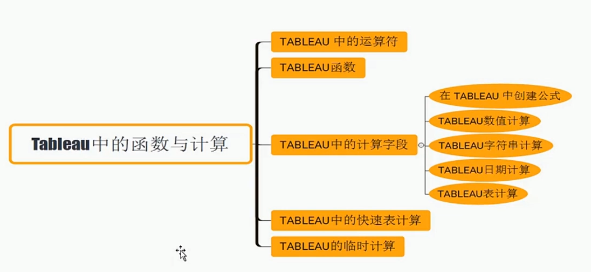1、Tableau中的运算符

运算符是一个符号，通知编译器执行特定的数学或逻辑操作，Tableau有多个运算符用于创建计算字段和公式，可用的运算符的详细信息和操作的顺序（优先级）如下：

运算符类型：常规运算符、算术运算符、关系运算符、逻辑运算符

（1）常规运算符

下表显示了Tableau支持的常规运算符。这些运算符对数字、字符和日期数据类型起作用

运算符

描述
例子

它添加两个数字，

它连接两个字符串，

它增加了日期的天数

7+3 利润+销售额

\'abc\'+\'def\'=\'abcdef\'

#April 15,2004# + 15 =

#April 30,2004#

-(subtraction)

它减去两个数字

它从日期中减去天数

-(7+3) = -10

#April16,2004# - 15 =

#April1,2004#

（2）算术运算符

这些运算符仅对数字数据类型起作用

运算符
描述
例子
*(Multiplication)
数值乘法
23 * 2 =46
/(Division)
数字部
45/2 = 22.5
%(modulo)
数值分割提醒
13%2 = 1
^(power)
提升到权力
2 ^ 3 = 8
（3）比较运算符

这些运算符用于表达式，每个运算符比较两个数字、日期或字符串，并返回布尔值（TRUE或FALSE）

运算符
描述
例子

2 ^ 3 = 8…

（此时=不是赋值等号）

比较两个数字或两个字符串或两个日期相等。返回布尔值TRUE或FALSE

\'Hello\'=\'Hello\'

S = 15/3

! = 或（不等于）
比较两个数字或两个字符串或两个日期不相等。返回布尔值TRUE，如果他们返回FALSE

\'好\'\'坏\'

18！ = 37/2

>（大于）

等等

比较两个数字或两个字符串或两个日期，其中第一个参数大于秒。返回FALSE或TRUE

[利润] > 2000

[Category] > \'Q\'

[发货日期] >= April 1,2004#

比较两个数字或两个字符串或两个日期，其中第一个参数小于秒。返回FALSE或TRUE

[利润]

[类别]

[发货日期]

（4）逻辑运算符

这些运算符用于其结果为布尔值的表达式，给出输出为TRUE或FALSE

运算符
描述
例子
AND
如果存在于AND运算符两侧的表达式或布尔值被计算为TRUE，则结果为TRUE。 否则结果为FALSE。
[发货日期]> #April 1，2012＃AND [Profit]> 10000
OR
如果存在于AND运算符两侧的任何一个或两个表达式或布尔值被计算为TRUE，则结果为TRUE。 否则结果为FALSE。
[发货日期]> #April 1，2012＃OR [Profit]> 10000
NOT
此运算符否定其后存在的表达式的布尔值。
NOT [Ship Date]> #April 1，2012＃
（5）优先级

① -(negate)      ② ^(power)     ③ *,/,%      ④ +，-      ⑤ ==，>，=

2、函数

常见内置函数：数字函数、字符串函数、日期函数、逻辑函数、聚合函数

（1）数字函数

数字函数
功能
描述
例子
CEILING(number)
将数字四舍五入为等于或大于值的最接近的整数

CEILING(2.145) = 3

FLOOR(数字)
将数字舍入为值相等或更小的最近整数
FLOOR(3.1415) = 3
ROUND(数字，[小数])
将数字四舍五入到指定的位数
ROUND(3.14152,2) = 3.14
POWER(基数，次方)
将数字提高到指定的地方
POWER(5,3) = 125
ABS(数字)
取绝对值
ABS(-10)
ACOS(number)
返回给定数字的反余弦，结果以弧度表示
ACOS(-1) = 3.14159265358979
ASIN(number)
返回给定数字的反正弦，结果以弧度表示
ASIN(1) = 1.5707963267949
ATAN(number)
返回给定数字的反正切，结果以弧度表示
ATAN(180) = 1.5652408283942
ATAN2(y number,x number)
返回两个给定数字（x和y）的反正切，结果以弧度表示
ATAN2(2，1) = 1.10714

COS(number)；

COT(number)

返回角度的余弦，以弧度为单位指定角度；

返回角度的余切，以弧度为单位指定角度

COS(PI()/4) = 0.707106781186548;

COT(PI()/4) = 1

DEGREES(number)
将以弧度表示的给定数字转换为度数
DEGREES(PI()/4) = 45.0
DIV(整数1，整数2)
返回将整数1除以整数2的除法运算的整数部分
DIV(11,2) = 5
EXP(number)
返回e的给定数字次幂

EXP(2) = 7,389

EXP(-[Growth Rate]*[Time])

LN(number)
返回数字的自然对数。如果数字小于或等于0，则返回null

LOG(number [,base])
返回数字以给定底数为底的对数，如果省略了底数值，则使用底数10

MAX(number.number)
返回两个参数（必须是同种类型）中的较大值。如果任一参数为null，则返回null，MAX也可应用于聚合计算中的单个字段

MAX(4,7)

MAX(Sales,Profit)

MAX(First Name,[Last Name])

MIN(number,number)
返回两个参数（必须是同种类型）中的较小值。如果任一参数为null，则返回null，MAX也可应用于聚合计算中的单个字段

MIN(4,7)

MIN(Sales,Profit)

MIN(First Name,[Last Name])

PI()
返回数字常量pi:3.14159

POWER(number,power)
计算数字的指定次幂

POWER(5,2) = 52 = 25

POWER(Temperature,2)

也可以使用^符号：

5^2 = POWER(5,2) = 25

将给定数字从度数转换为弧度
SQUARE(number)
返回数字的平方
SQUARE(5) = 25
如何使用：在列/行里都可以，直接输入计算函数，右键选择维度查看计算结果

（2）字符串函数

功能
描述
例子
ASCII（string）
返回string的第一个字符的ASCII代码
ASCII('A') = 65
CHAR(number)
返回通过ASCII代码number编码的字符
CHAR(65) = 'A'

Contains(string,substring)

(区分大小写)

如果给定字符串包含指定子字符串，则返回true
CONTAINS("Calculation","alcu") = true

ENDSWITH(string, substring)

（区分大小写）

如果给定字符串以指定子字符串结尾，则返回true，会忽略尾随空格
ENDSWITH("Tableau","leau") = true
FIND(string,substring,[start])
返回substring在string中的索引位置，如果未找到substring，则返回0。如果添加了可选参数start，则函数会忽略在索引位置start之前出现的任何substring实例,字符串中的第一个字符为位置1

FIND("Calculation","alcu") = 2

FIND("Calculation","a",3) = 7

FIND("Calculation","aswd") = 0

FINDNTH(string,substring,

occurrence)

返回指定字符串内的第n个子字符串的位置，其中n由occurrence参数定义
FINDNTH("Calculation","a",2) = 7
LEFT(string,number)
返回字符串最左侧一定数量的字符
LEN(string)
返回字符串长度

LOWER(string)

UPPER(string)

返回string，其所有字符为小写;

返回string，其所有字符为大写;

LOWER("ProductVersion") = "productversion"

LTRIM(string)；

RTRIM(string)；

TRIM(string)

返回移除了所有前导空格的字符串（只去前，不去后）;

返回移除了所有尾随空格的字符串;

返回移除了前导和尾随空格的字符串。

LTRIM("             cij   dcjd         ") = “cij   dcjd         ”

RTRIM("             cij   dcjd         ") = “             cij   dcjd”

len(TRIM(" Hello ")) = 5

MAX(a,b)

（从左向右依次比较，先比较第一个，比不出依次往后）

返回a和b(必须为相同类型)中的较大值，此函数常用于比较数字，但也对字符串有效。对于字符串，MAX查找数据库为该列定义的排序序列中的最高值。如果任一参数为Null，则返回Null
MAX("APPLE","banana")= "banana"
MID(string,start,[length])
返回从索引位置start开始的字符串。字符串中第一个字符的位置为1.如果添加了可选参数length,则返回的字符串仅包含该数量的字符。

MID("Calculation",2) = "alculation"

MID("Calculation",2,5) = "alcul"

MIN(a,b)
返回a和b(必须为相同类型)中的较小值，此函数常用于比较数字，但也对字符串有效。对于字符串，MIN查找排序序列中的最低值，如果任一参数为Null，则返回Null
MIN("APPLE","banana")= "APPLE"

REPLACE(string,substring,

replacement)

在string中搜索substring并将其替换为replacement。如果未找到substring，则字符串保持不变
REPLACE("V8.5","8.5","9.0") = "V9.0"
RIGHT(string,number)
返回string中最右侧一定数量的字符
RIGHT("Calculation",4) = "tion"
SPACE(number)
返回由指定number个重复空格组成的字符串
SPACE("1") = " "
SPLIT(string,delimiter,token number)

返回字符串中的一个子字符串，并使用分隔符字符将字符串分为一系列标记.

字符串将被解释为分隔符和标记的交替序列。因此，对于字符串abc-def-i-j，分隔符字符为“-”，标记为abc、def、i、j，将这些标记想像为标记1至4.SPLIT将返回与标记编号对应的标记。如果标记编号为正，则从字符串的左侧开始计算标记，如果标记编号为负，则从右侧开始计算标记。

SPLIT("a-b-c-d","-",2) = "b"
STARTSWITH(string,substring)
如果string以substring开头，则返回true，会忽略前导空格
STARTSWITH("Joker","Jo") = true
（3）日期函数

Tableau提供了多种日期函数。许多示例将#符号用于日期表达式。此外，许多日期函数还使用date_part，它是一个常量字符串参数，可以使用的有效date_part值为：

有效date_part值
date_part
值
'year'
四位数年份
'quarter'
1-4
‘month’
1-12 或 ”January“、”February“等
”dayofyear“
一年中的第几天：1月1日为1、2月1日为32，以此类推
”day“
1-31
”weekday“
1-7 或 ”Sunday“、”Monday“等
”week“
1-52
”hour“
0-23
”minute“
0-59
”second“
0-60
日期函数
返回指定日期，该日期的指定date_part中添加了指定的数字interval

该表达式会向日期#2004-04-15#添加三个月

DATEDIFF(date_part,date1,date2,[start_of_week])

返回date1与date2之差（以date_part的单位表示）；

start_of_week参数(可用于指定哪一天是一周的第一天）是可选的。可能的值为”monday“、”tuesday“等。如果省略，一周的开始由数据源确定。

DATEDIFF("week",#2013-09-22#,#2013-09-24#,"monday") = 1

DATEDIFF('week',#2013-09-22#,#2013-09-24#,'sunday') = 0

DATENAME(date_part,date,[start_of_week])

以字符串的形式返回date的date_part；

start_of_week参数(可用于指定哪一天是一周的第一天）是可选的。可能的值为”monday“、”tuesday“等。如果省略，一周的开始由数据源确定。

DATENAME('Year',#2004-04-15#) = "2004"

DATENAME('month',#2004-04-15#) = "April"

DATEPART(date_part,date,[start_of_week])

以整数的形式返回date的date_part；

start_of_week参数(可用于指定哪一天是一周的第一天）是可选的。可能的值为”monday“、”tuesday“等。如果省略，一周的开始由数据源确定。

注：当date_part为工作日时，会忽略start_of_week参数。这是因为Tableau依赖固定工作日顺序来应用偏移

DATEPART('year',#2004-04-15#) = 2004

DATEPART('month',#2004-04-15#) =4

DATETRUNC(date_part,date,[start_of_week])

按date_part指定的准确度截断指定日期。此函数返回新日期。例如，以月份级别截断处于月份中间的日期时，此函数返回当月的第一天。

start_of_week参数(可用于指定哪一天是一周的第一天）是可选的。可能的值为”monday“、”tuesday“等。如果省略，一周的开始由数据源确定。

DATETRUNC('quarter',#2004-08-15#) = 2004-07-01 12:00:00 AM

DATETRUNC('month',#2004-04-15#) = 2004-04-01 12:00:00 AM

DAY/MONTH/YEAR(date)
以整数的形式返回给定日期的天/月/年
DAY(#2004-04-12#) = 12
ISDATE(string)
如果给定字符串为有效日期，则返回true
ISDATE("2001-1-23")
MAKEDATE(year,month,day)

返回一个依据指定年份、月份和日期构造的日期值；

可用于Tableau数据提取，检查在其他数据源中的可用性

MAKEDATE(2004,4,15) = #April 15,2004#
MAKEDATETIME(date,time)
返回合并了date和time的datetime。日期可以是date、datetime或string类型。时间必须是datetime。此功能仅适用于与MySQL兼容的连接（对于Tableau，除MySQL之外，还有Amazon Aurora 和 Amazon Aurora）

MAKEDATETIME(#1890-12-30#,#07:59:00#) = #12/30/1899  7:59:00 AM#

yyyy/m/d  hh:nn:ss

NOW()

返回当前日期和时间。

返回值因连接的特性而异：

对于实时、未发布的连接，NOW返回数据服务器时间；

对于实时、已发布的连接，NOW返回数据服务器时间；

对于未发布的数据提取，NOW返回本地系统时间；

对于发布的数据提取，NOW返回Tableau Server数据引擎的本地时间。如果在不同时区中有多台工作计算机，这可能会产生不一致的结果。

NOW() = 2019/8/21 17:10:01

TODAY()
返回当前日期
TODAY() = 2019/8/21

（4）聚合函数

功能
描述
AVG(expression)
返回表达式中所有值得平均值。AVG只能与数字字段一起使用，空值被忽略
COUNT(expression)
返回组中的项目数，不计算空值
COUNTD(expression)
返回组中不同项目的数量，不对Null值计数
MEDIAN(expression)
返回所有记录的表达式的中位数，中值只能与数字字段一起使用，空值被忽略
STDEV(expression)
返回基于样本总体的给定表达式中所有值的统一标准偏差
MIN,MAX,SUM

（5）类型转换函数

计算中任何表达式的结果都可以转换为特定数据类型。转换函数为 STR()、DATE()、DATETIME()、INT() 和 FLOAT()。例如，如果要将浮点数（如 3.14）转换为整数，则可以编写 INT(3.14)。结果为 3（这是整数）。下面介绍了转换函数。

可以将布尔值转换为整数、浮点数或字符串，但不能将其转换为日期。True 为 1、1.0 或“1”，而 False 为 0、0.0 或“0”。Unknown 映射到 Null。

功能
描述
例子
DATE(expression)
在给定数字、字符串或日期表达式的情况下返回日期。

DATE([Employee Start Date])

DATE("4/15/2004")=
2004/4/15

DATE("April 15, 2004") = #April 15, 2004# DATE(#2006-06-15 14:52#) = #2006-06-15#

注：第二个和第三个，引号不可省

DATETIME(expression)
在给定数字、字符串或日期表达式的情况下返回日期时间。
DATETIME(“April 15, 2005 07:59:00”) = April 15, 2005 07:59:00
DATEPARSE(format, string)
将字符串转换为指定格式的日期时间。是否支持某些区域设置特定的格式由计算机的系统设置确定。数据中出现的不需要解析的字母应该用单引号 (' ') 引起来。对于值之间没有分隔符的格式（如 Mmddyy），请验证它们是否按预期方式解析。该格式必须是常量字符串，而非字段值。如果数据与格式不匹配，此函数将返回 Null。

DATEPARSE ("dd.MMMM.yyyy", "15.April.2004") = #April 15, 2004#

DATEPARSE ("h'h' m'm' s's'", "10h 5m 3s") = #10:05:03#

FLOAT(expression)
将其参数转换为浮点数。

FLOAT(3) = 3.000

FLOAT([Age]) 将 Age 字段中的每个值转换为浮点数。

INT(expression)
将其参数转换为整数。对于表达式，此函数将结果截断为最接近于 0 的整数。

INT(8.0/3.0) = 2

INT(4.0/1.5) = 2

INT(0.50/1.0) = 0

INT(-9.7) = -9

字符串转换为整数时会先转换为浮点数，然后舍入。

STR(expression)
将其参数转换为字符串。
STR([Age]) 会提取名为 Age 的度量中的所有值，并将这些值转换为字符串。
（6）逻辑函数

①AND

定义：对两个表达式执行逻辑合取计算

语法：IF <expr1> AND <expr2> THEN <then> END

例子：IF (ATTR([Market]) = "Africa" AND SUM([Sales]) > [Emerging Threshold] )THEN "Well Performing"

②CASE

定义：执行逻辑测试并返回相应的值。CASE函数可评估expression，并将其与一系列值（value1、value2等）比较，然后返回结果。遇到一个与expression匹配的值时，CASE返回相应的返回值。如果未找到匹配值，则使用默认返回表达式。如果不存在默认返回表达式并且没有任何值匹配，则会返回Null

语法：CASE <expression> WHEN <value1> THEN <return1> WHEN <value2> THEN <return2> ... ELSE <default return> END

实例：CASE [Region] WHEN 'West' THEN 1 WHEN 'East' THEN 2 ELSE 3 END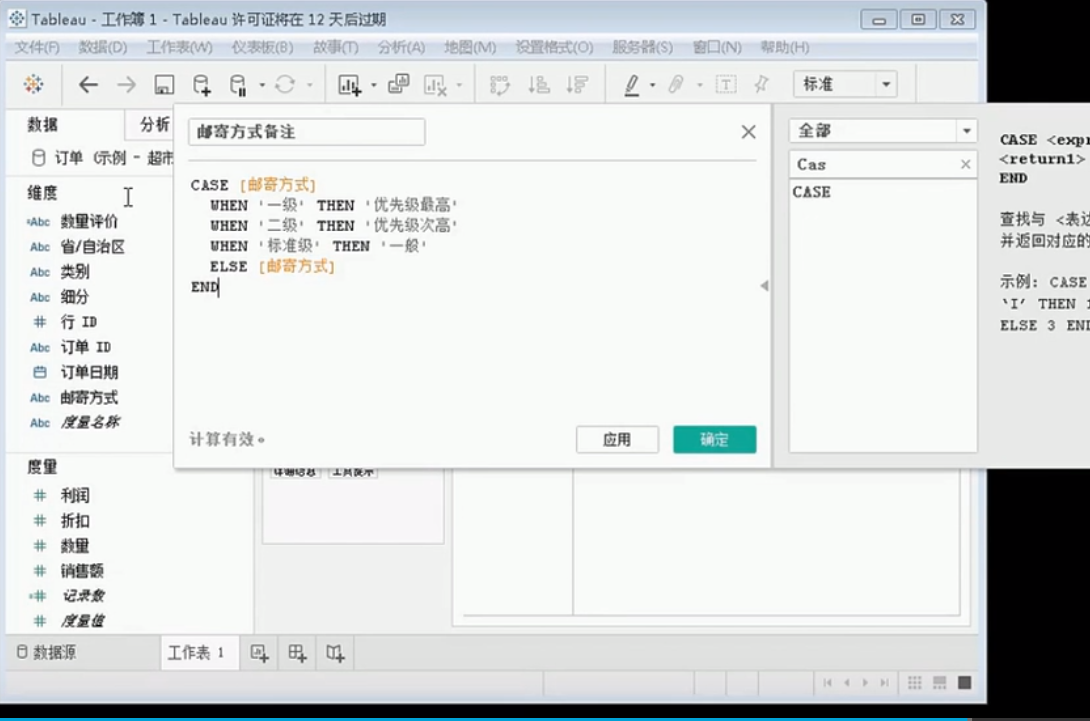③ELSE

定义：测试一系列表达式，同时为第一个为 true 的 <expr> 返回 <then> 值。

语法：IF <expr> THEN <then> ELSE <else> END

示例：If [Profit] > 0 THEN 'Profitable' ELSE 'Loss' END

④ELSEIF

定义：测试一系列表达式，同时为第一个为 true 的 <expr> 返回 <then> 值。

语法：IF <expr> THEN <then> [ELSEIF <expr2> THEN <then2>...] [ELSE <else>] END

示例：IF [Profit] > 0 THEN 'Profitable' ELSEIF [Profit] = 0 THEN 'Breakeven' ELSE 'Loss' END

⑤END

定义：测试一系列表达式，同时为第一个为 true 的 <expr> 返回 <then> 值。必须放在表达式的结尾。

语法：IF <expr> THEN <then> [ELSEIF <expr2> THEN <then2>...] [ELSE <else>] END

示例：IF [Profit] > 0 THEN 'Profitable' ELSEIF [Profit] = 0 THEN 'Breakeven' ELSE 'Loss' END

⑥IF

定义：测试一系列表达式，同时为第一个为 true 的 <expr> 返回 <then> 值。

语法：IF <expr> THEN <then> [ELSEIF <expr2> THEN <then2>...] [ELSE <else>] END

示例：IF [Profit] > 0 THEN 'Profitable' ELSEIF [Profit] = 0 THEN 'Breakeven' ELSE 'Loss' END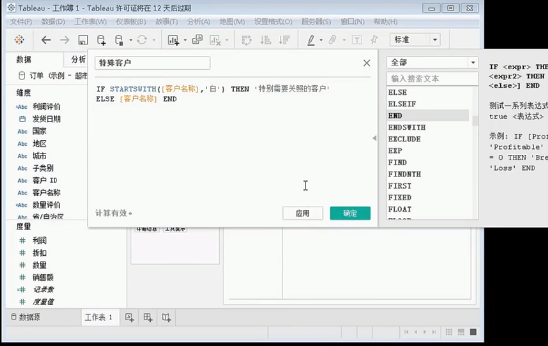⑦IFNULL

定义：如果 <expr1> 不为 null，则返回该表达式，否则返回 <expr2>。

语法：IFNULL(expr1, expr2)

示例：IFNULL([Profit], 0)

⑧IIF

定义：检查某个条件是否得到满足，如果为 TRUE 则返回一个值，如果为 FALSE 则返回另一个值，如果未知，则返回可选的第三个值或 NULL。

语法：IIF(test, then, else, [unknown])

示例：IIF([Profit] > 0, 'Profit', 'Loss')

⑨ISDATE

定义：如果给定字符串为有效日期，则返回 true。

语法：ISDATE(string)

示例：ISDATE("2004-04-15") = True

⑩ISNULL

定义：如果表达式未包含有效数据 (Null)，则返回 true。

语法：ISNULL(expression)

示例：ISNULL([Profit])

11 MAX

定义：返回单一表达式所有记录中的最大值，或返回每条记录两个表达式中的最大值。

语法：MAX(expression) 或 Max(expr1, expr2)

示例：MAX([Sales])

12 MIN

定义：返回单一表达式所有记录中的最小值，或返回每条记录两个表达式中的最小值。

语法：MIN(expression) 或 MIN(expr1, expr2)

示例：MIN([Profit])

13 NOT

定义：对两个表达式执行逻辑非运算。

语法：IF NOT <expr> THEN <then> END

示例：IF NOT [Profit] > 0 THEN "Unprofitable" END

或者

定义：对两个表达式执行逻辑析取操作。

语法：IF <expr1> OR <expr2> THEN <then> END

示例：IF [Profit] < 0 OR [Profit] = 0 THEN "Needs Improvement" END

14 THEN

定义：测试一系列表达式，同时为第一个为 true 的 <expr> 返回 <then> 值。

语法：IF <expre> THEN <then> [ELSEIF ,expr2> THEN <then2>...] [ELSE <else>] END

示例：IF [Profit] > 0 THEN 'Profitable' ELSEIF [Profit] = 0 THEN 'Break even' ELSE 'unprofitable' END

15 WHEN

定义：查找第一个与 <expr> 匹配的 <value>，并返回对应的 <return>。

语法：CASE <expr> WHEN <Value1> THEN <return1> ... [ELSE <else>] END

示例：CASE [RomanNumberal] WHEN 'I' THEN 1 WHEN 'II' THEN 2 ELSE 3 END

16 ZN

定义：如果 <expression> 不为 null，则返回该表达式，否则返回零。

语法：ZN(expression)

示例：ZN([Profit])

3、在tableau中创建公式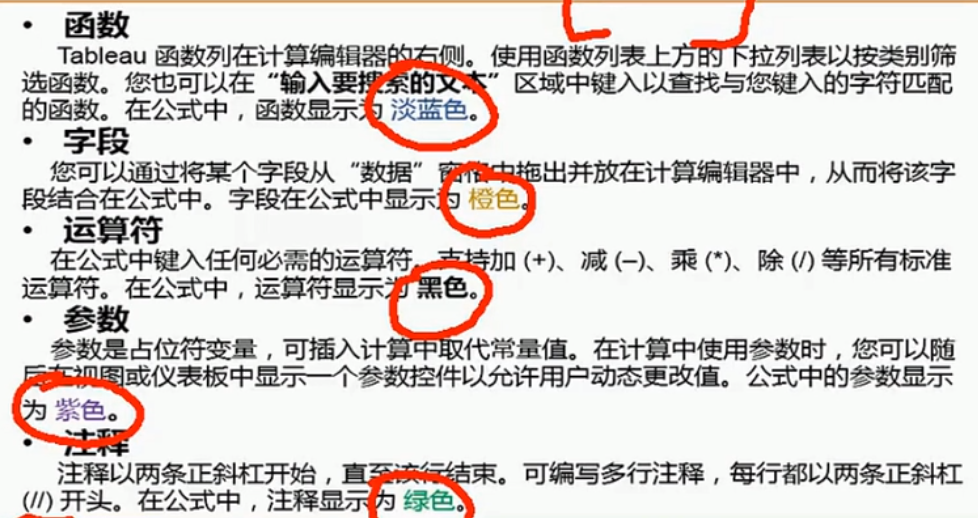4、Tableau数值计算（1）计算字段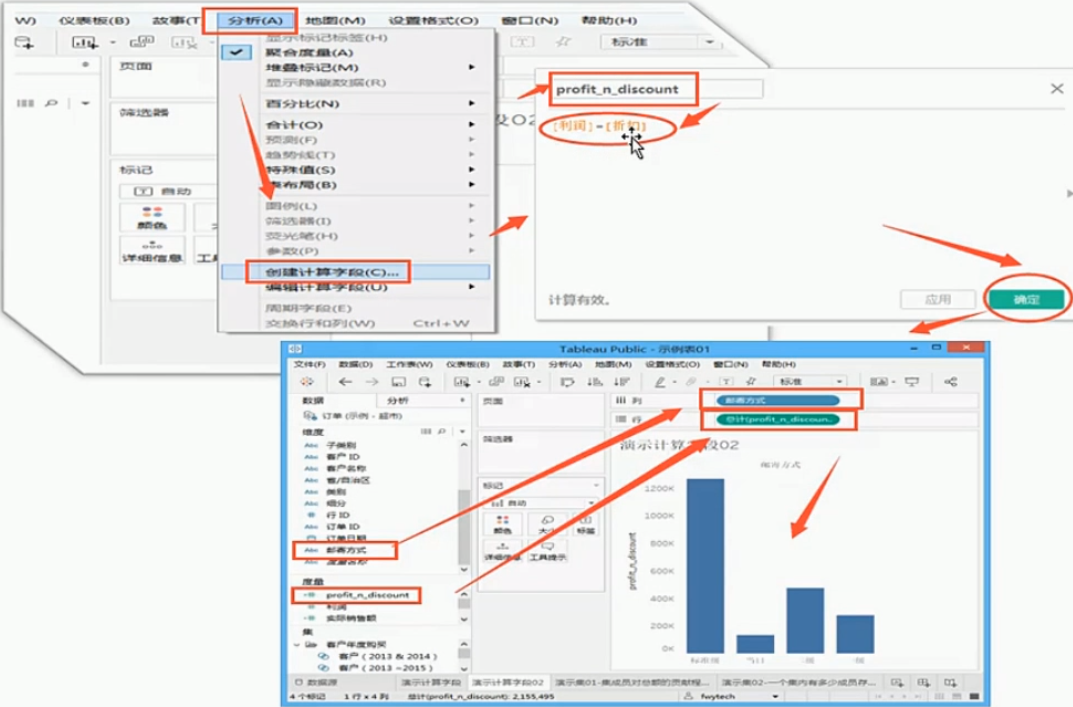（2）聚合字段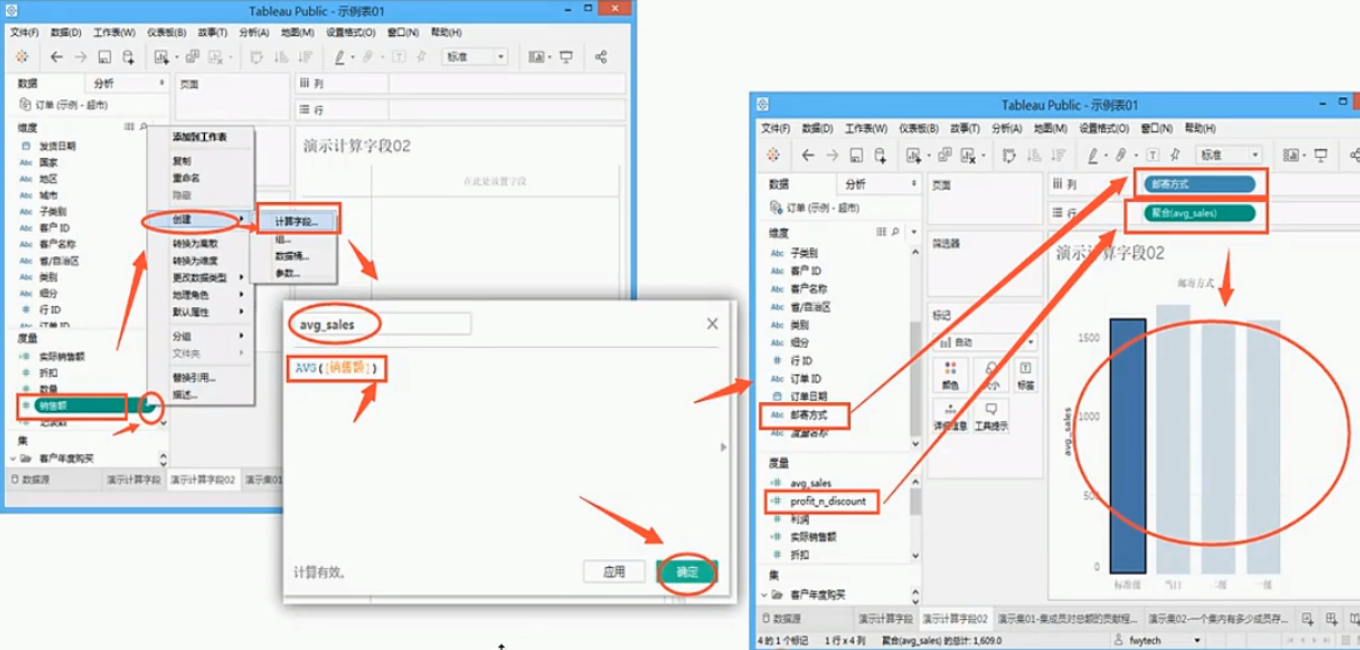5、Tableau字符串计算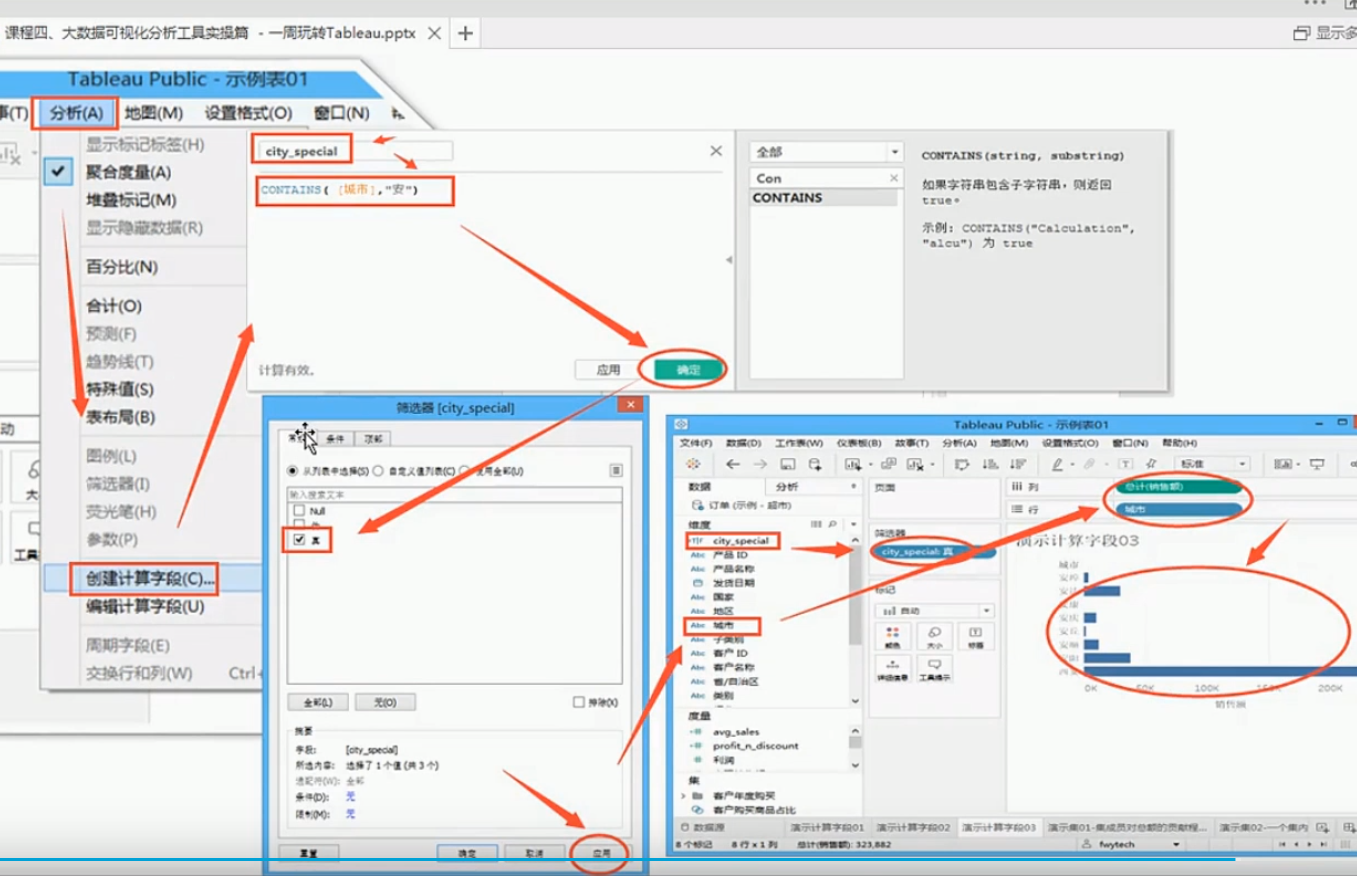6、Tableau日期计算

示例：了解每种商品的【订单日期】与【发货日期】的时间总间隔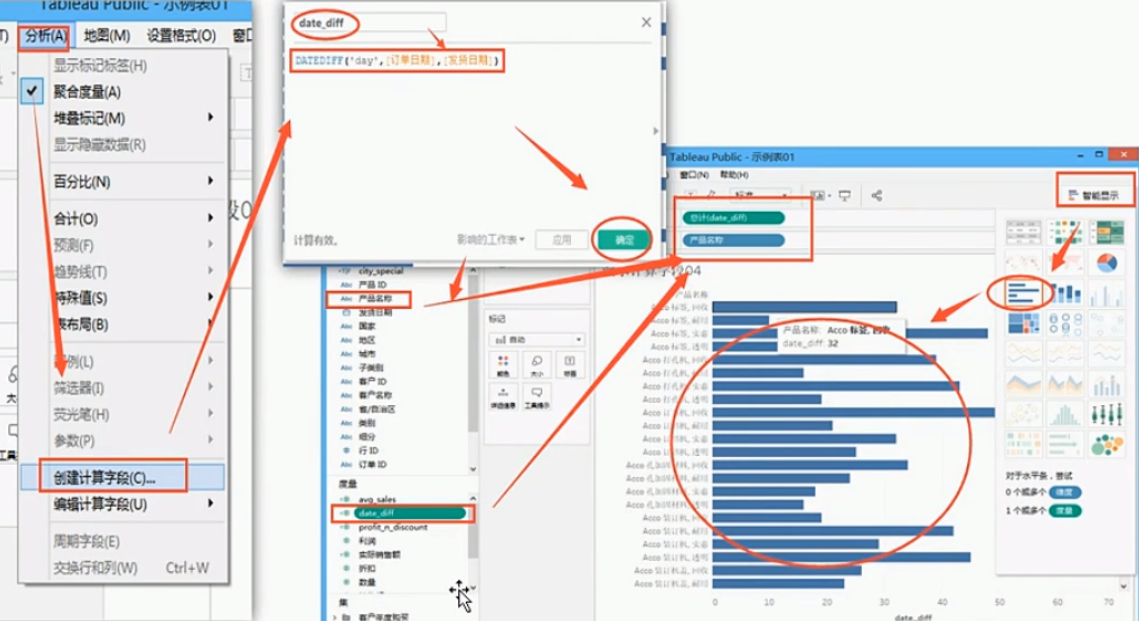7、表计算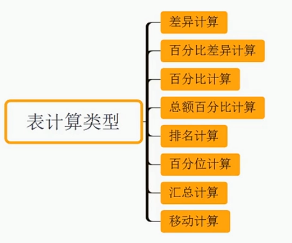（1）表计算01-差异计算

原始图表：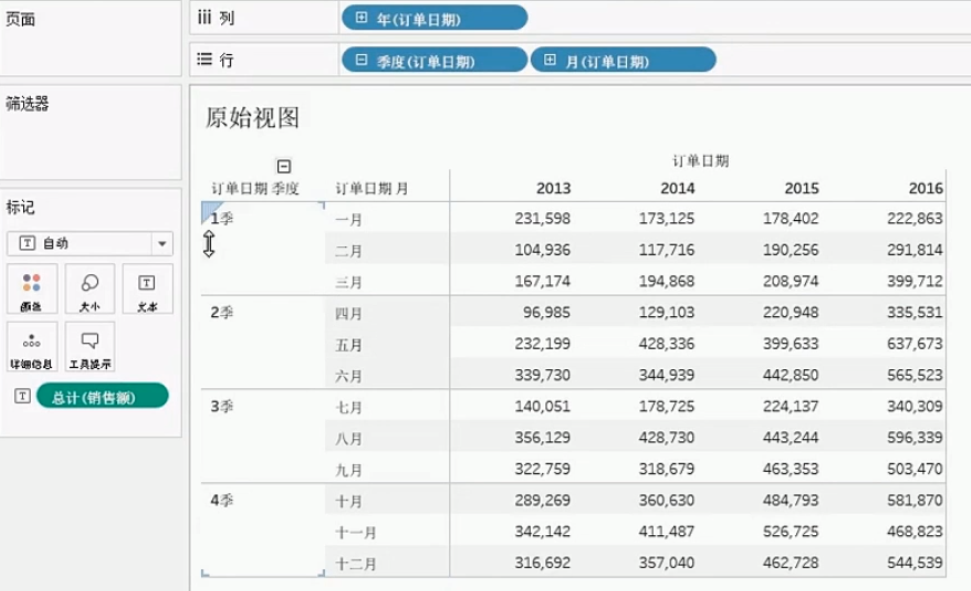①居中：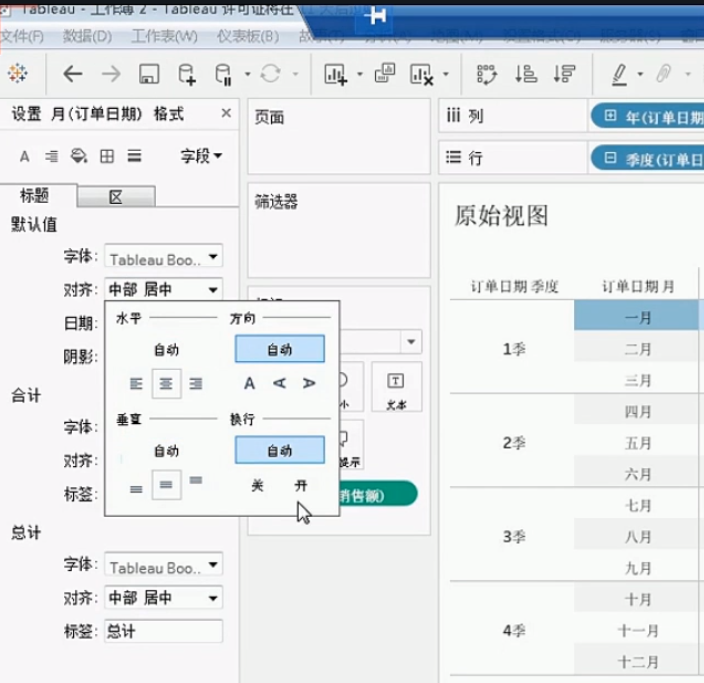②设置货币格式③差异计算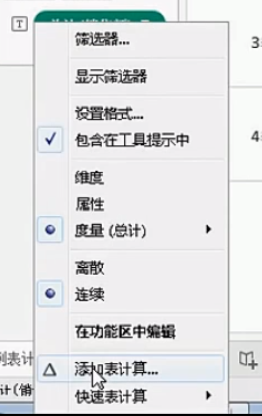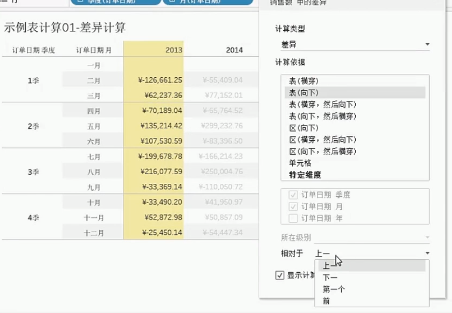（2）百分比差异计算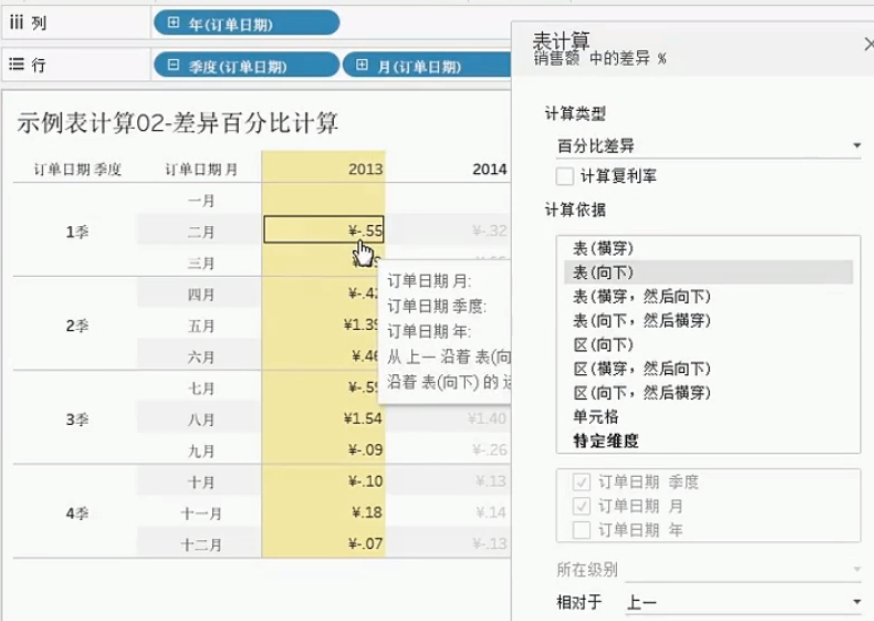（3）百分比计算

：”百分比“表计算会将值计算为某个其他值的百分比 - 通常计算为表中前一个值的百分比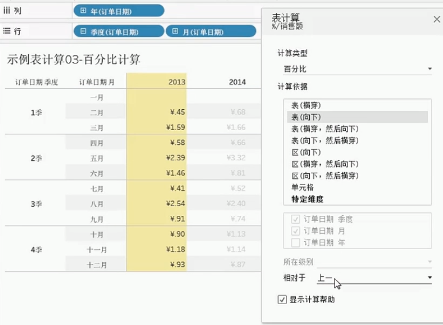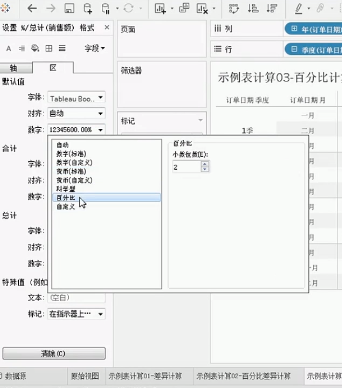（4）总额百分比计算

对于视图中的每个标记，“总额百分比”表计算会将值计算为当前分区中所有值的百分比。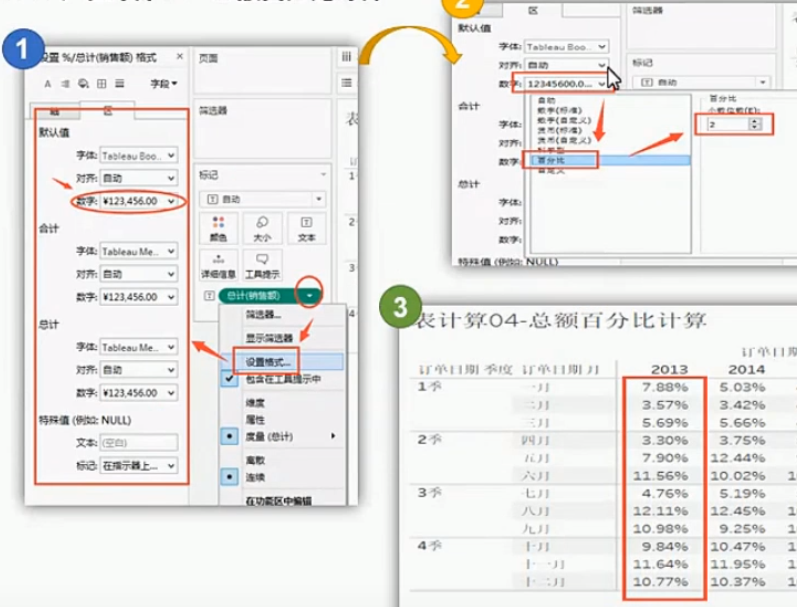（5）表计算05-排名计算

“排名”表计算将计算分区中每个值的排名（6）表计算06-百分位计算

“百分位”表计算将在分区中计算每个值的百分位排名。

对于表计算，分配给最低排名值得百分位为0，分配给最高排名值的百分位为100（默认升序），系统会按排名为所有值分配百分位，因此，对于1、3、22、67这一组的四个值，百分位将分别为0%、33%、67%和100%。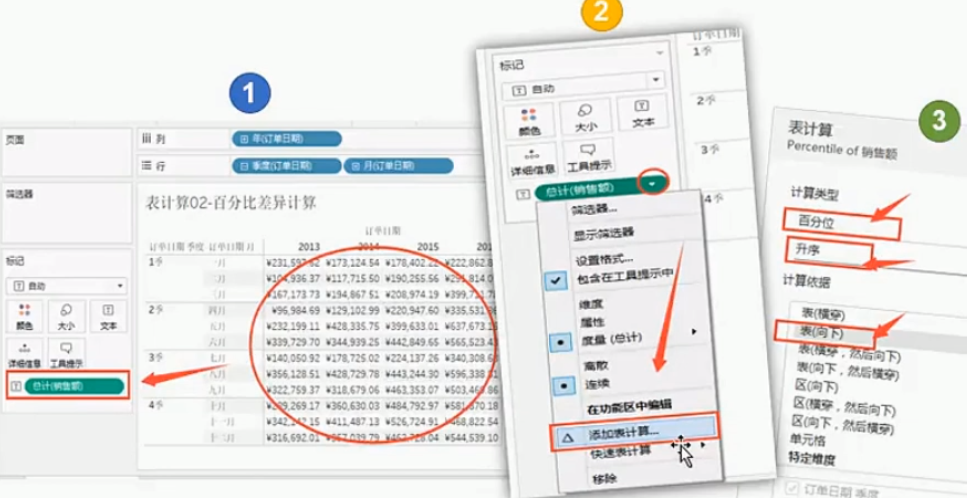（7）汇总计算

对于“汇总”和“移动计算”表计算，您可以选择转换两次值，以获得您想要的结果-即除了添加主要计算之外再添加从属表计算。例如：可以添加一个初始表计算来计算每一年内每个月的销售汇总，然后添加从属计算一年与下一年同期相比每个月的年基差异百分比。

①汇总计算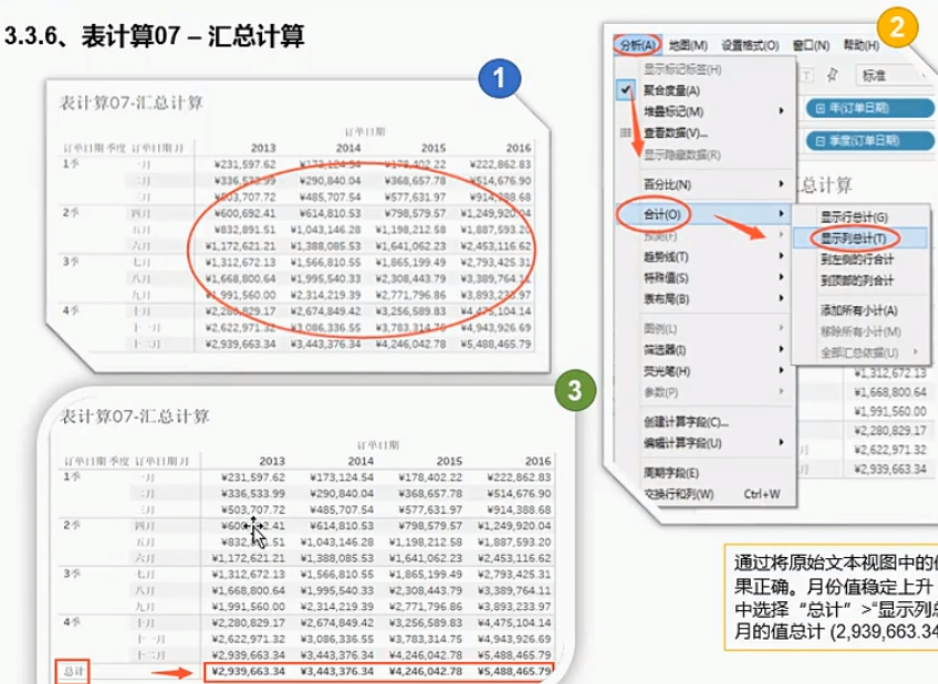②从属计算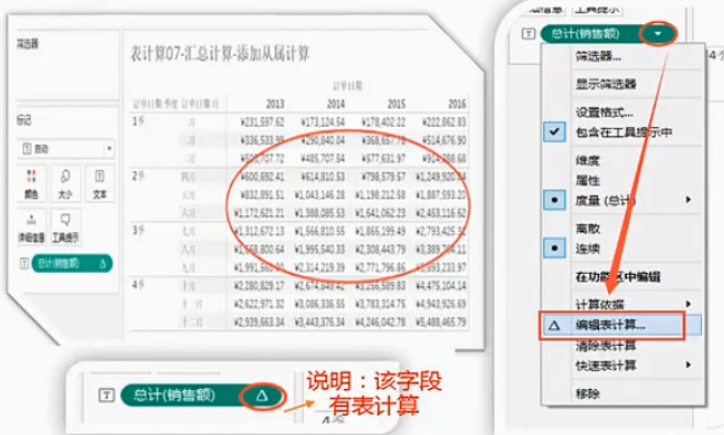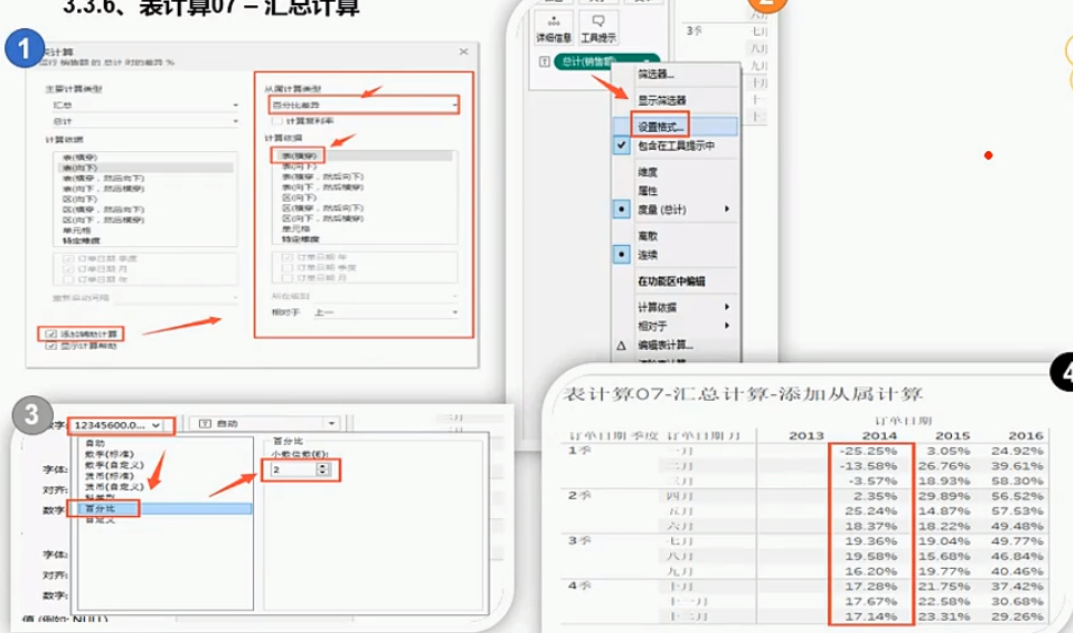（8）表计算08-移动计算

“移动计算”表计算（有时称为 滚动计算）会对当前值之前和/或之后指定数目的值执行聚合（总计值、平均值、最小值或最大值）来确定视图中的标记值。移动计算通常用于平滑短期数据波动，这样可以查看长期趋势。例如，对于证券数据，市场每天都在波动，很难通过每日的上升和下降把握全局，可以使用移动计算来定义一系列值通过所选聚合进行汇总。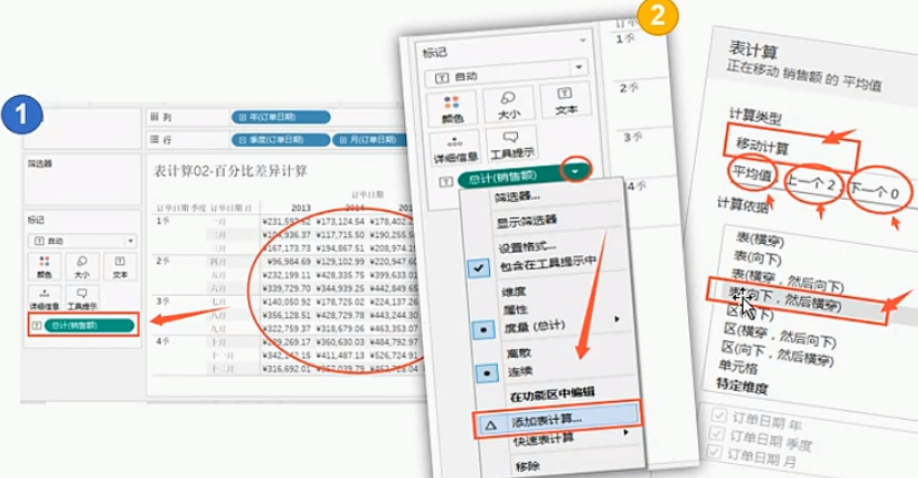（9）快速表计算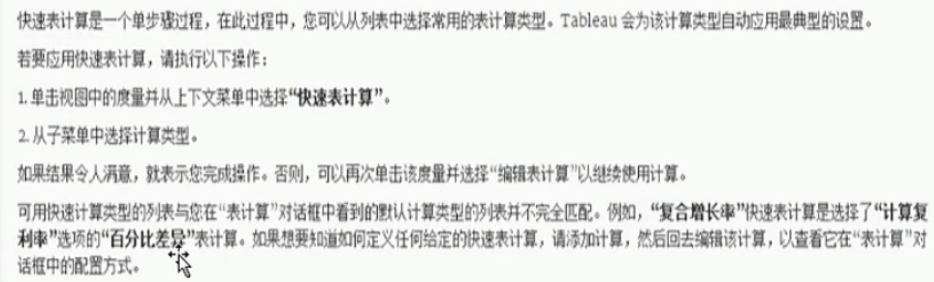8、临时计算

（1）临时计算是在处理视图中功能区上的字段时可创建和更新的计算；临时计算也称为调用类型输入计算或内联计算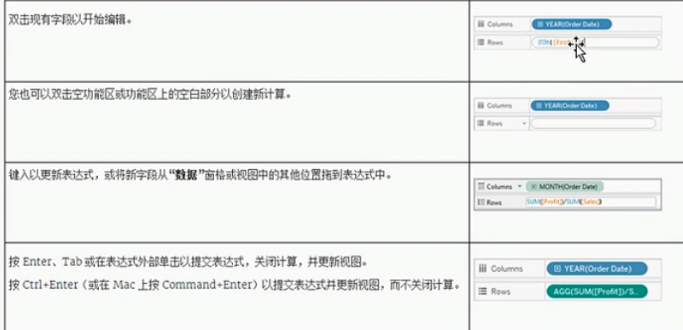（2）多行临时计算

键入临时计算时，可以使用Shift+Enter另起一行。但是，临时计算中只有当前行始终可见，因此对于正在查看或编辑计算并且无法得知计算中包含多行的任何人来说，这可能会造成混淆。

多行临时计算的第一行可能是用作计算标题的备注。这是提交计算之后功能区唯一可见的行。

//City  and  State       //代表注释

[City] +' , ' + [State]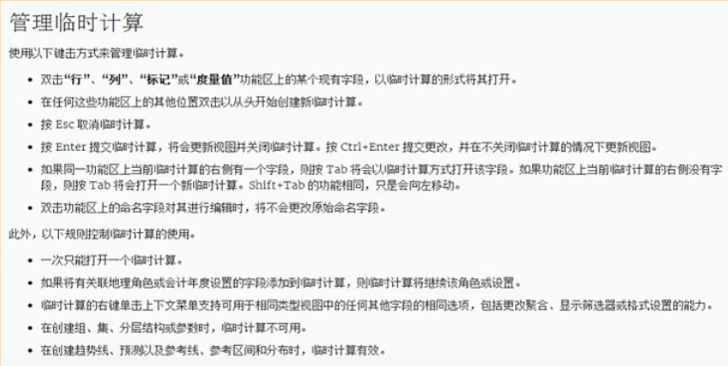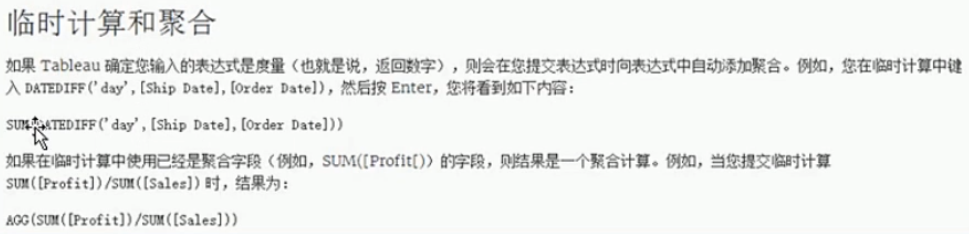展开全文• logical函数（逻辑函数） logical(x)：x ~=0时，logical(x)=1；x = 0时，logical(x)=0 注意： 一·logical函数可以将矩阵转化为逻辑矩阵 例： a =  0 0 0 0 0 0 0 0 0 ...
logical函数（逻辑函数）

logical(x)：x ~=0时，logical(x)=1；x = 0时，logical(x)=0

注意：

一·logical函数可以将矩阵转化为逻辑矩阵

例：

a =

0         0         0         0         0         0         0         0         0         0
0         0         0         0         0         0         0         0         0         0
0         0         0         0         0         0         0         0         0         0
5.2853    9.1334    7.7491    2.6380    5.1325    9.4479    4.0391    0.1540    1.8896    4.3586
1.6565    1.5238    8.1730    1.4554    4.0181    4.9086    0.9645    0.4302    6.8678    4.4678
6.0198    8.2582    8.6869    1.3607    0.7597    4.8925    1.3197    1.6899    1.8351    3.0635
2.6297    5.3834    0.8444    8.6929    2.3992    3.3772    9.4205    6.4912    3.6848    5.0851
6.5408    9.9613    3.9978    5.7970    1.2332    9.0005    9.5613    7.3172    6.2562    5.1077
6.8921    0.7818    2.5987    5.4986    1.8391    3.6925    5.7521    6.4775    7.8023    8.1763
7.4815    4.4268    8.0007    1.4495    2.3995    1.1120    0.5978    4.5092    0.8113    7.9483

>> logical(a)

ans =

0     0     0     0     0     0     0     0     0     0
0     0     0     0     0     0     0     0     0     0
0     0     0     0     0     0     0     0     0     0
1     1     1     1     1     1     1     1     1     1
1     1     1     1     1     1     1     1     1     1
1     1     1     1     1     1     1     1     1     1
1     1     1     1     1     1     1     1     1     1
1     1     1     1     1     1     1     1     1     1
1     1     1     1     1     1     1     1     1     1
1     1     1     1     1     1     1     1     1     1
展开全文• 逻辑函数的简化方法是简化逻辑电路，用最少的电子器件实现这个逻辑函数。 如下的真值表，如果直接从真值表转换为逻辑电路图，需要8个与，一个或，但简化后只需要4个与，1个或。 ...
逻辑函数的简化方法是简化逻辑电路，用最少的电子器件实现这个逻辑函数。
如下的真值表，如果直接从真值表转换为逻辑电路图，需要8个与，一个或，但简化后只需要4个与，1个或。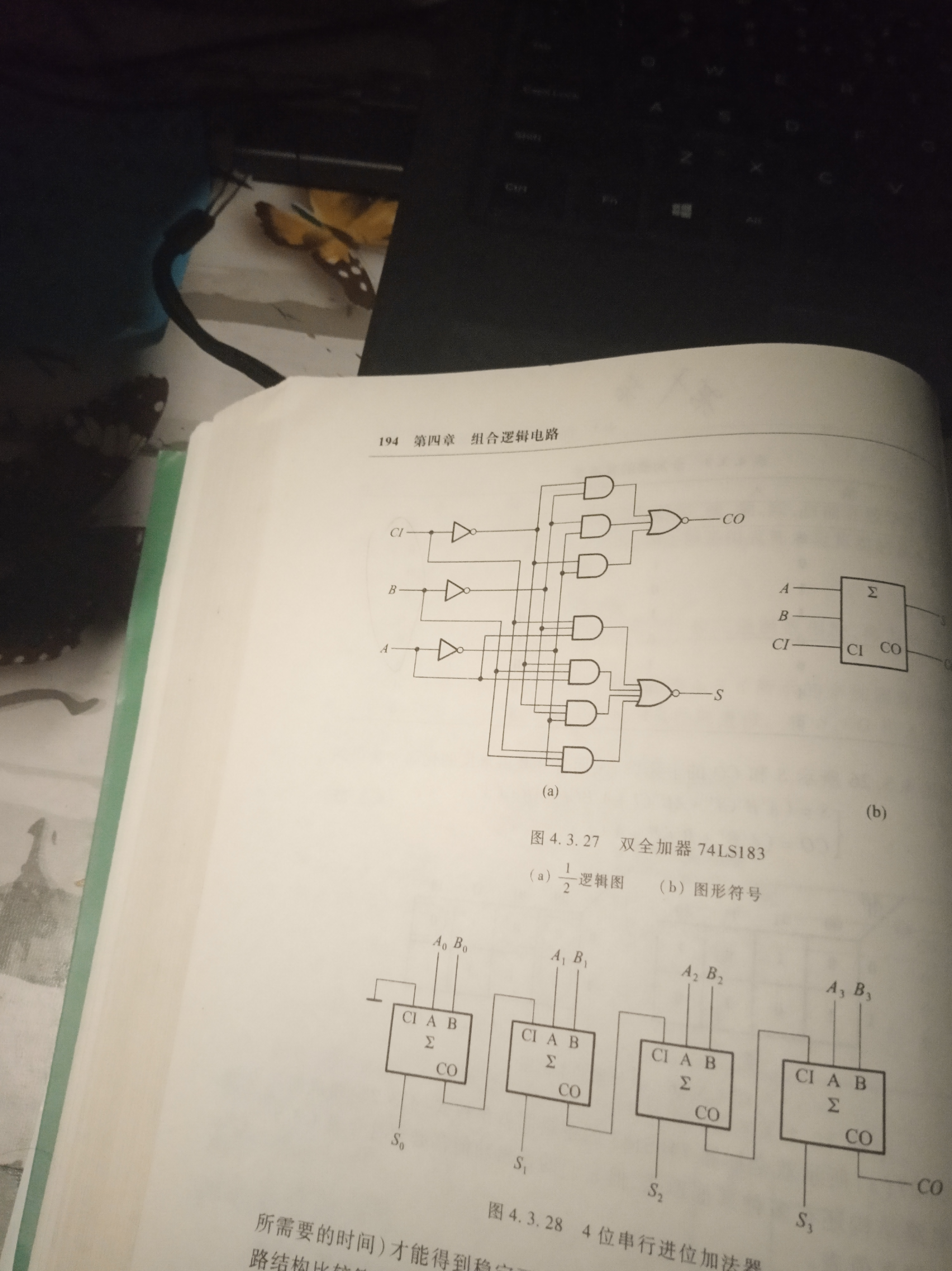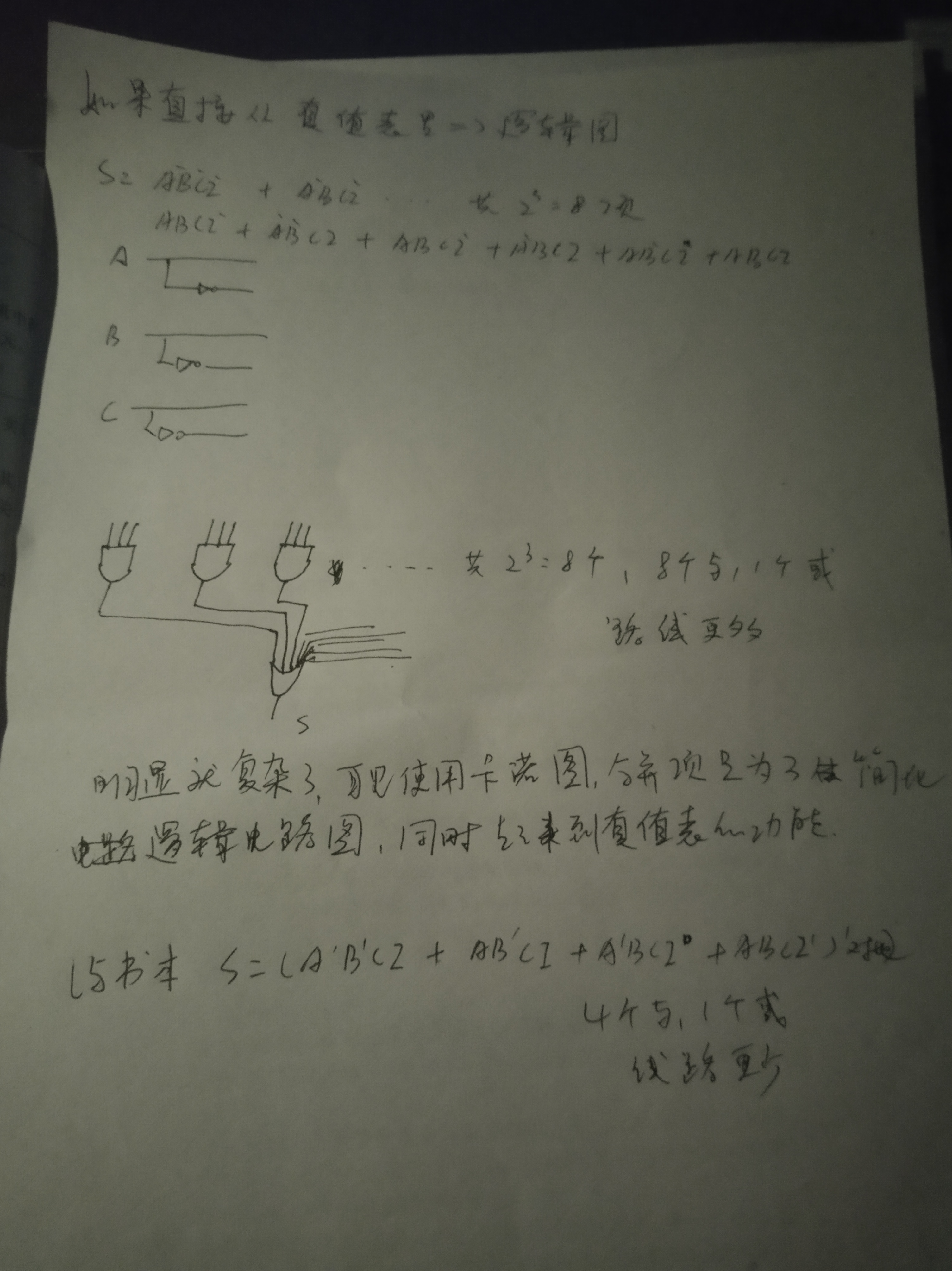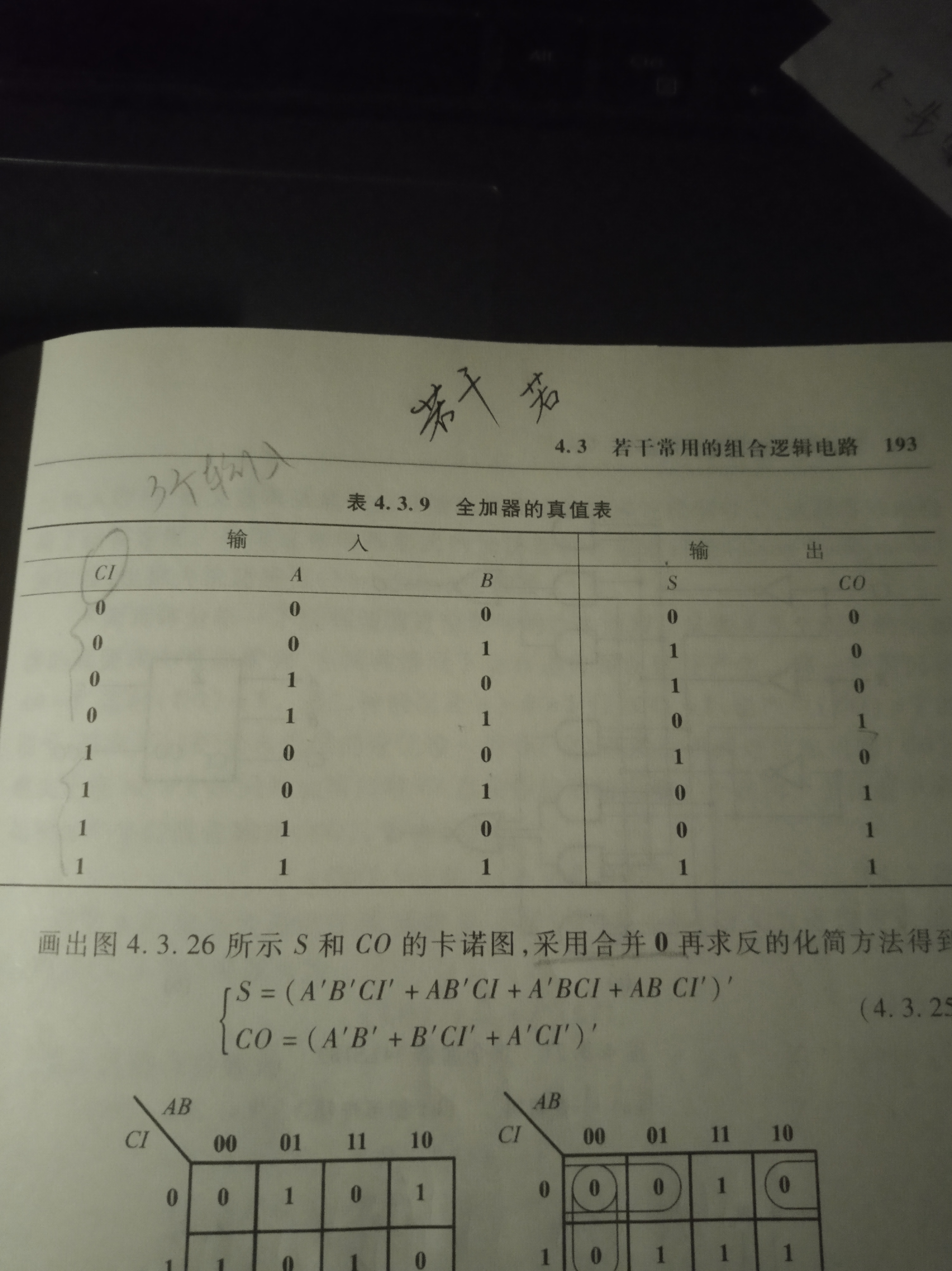展开全文• F能推出 原不直观存在于 函数依赖集F 中的函数依赖 α →→\to β,则成α→→\toβ被函数依赖集F逻辑蕴含 函数依赖的闭包 F+F+F^+: ​ 由关系模式R直观得到的函数依赖F所推出的所有隐含的或未隐含的(直观...数据库设计 属性闭包算法 无损分解
• 一、线性回归损失函数的两种解释线性回归的损失函数是平方损失函数，为什么使用平方的形式，参考：线性回归损失函数为什么要用平方形式，讲得很清楚。 在线性回归中，对于训练数据样本(xi,yi)(x_i,y_i)，我们有如下...线性回归
• 从微软网站上找到的关于 Excel 上 QUARTILE() 函数的计算逻辑，与分位值的常规计算方式略有差异，因此记录下来，供需要时参考：The following is the algorithm used to calculate QUARTILE():1) Find the kth ...excel 微软
• 逻辑功能中简单概括得出的逻辑函数，往往不是最简表达式，根据这样的非最简式来实现电路，系统会过于复杂，成本过高，同时，电路运行的安全性和可靠性也无法得到保障。 为了降低系统成本，提高工作可靠性，应在不...数字电子技术 函数
• ## 逻辑回归中的损失函数的解释

万次阅读 多人点赞 2018-06-05 18:19:11
逻辑回归是机器学习中的一个非常常见的模型， 逻辑回归模型其实仅在线性回归的基础上，套用了一个逻辑函数。 逻辑回归可以看做是两步，第一步和线性回归模型的形式相同，即一个关于输入x的线性函数： 第二步通过...
• MATLAB 语言中的逻辑函数 逻辑函数 函数意义 all 判断是否所有元素为非零值 any 判断是否存在一个元素为非零值 exist 查看变量或函数是否存在 find 找出向量或矩阵中非零元素...
• 根据条件计算值在了解了IF函数的使用方法后，我们再来看看与之类似的Excel提供的可根据某一条件来分析数据的其他函数。例如，如果要计算单元格区域中某个文本串或数字出现的次数，则可使用 COUNTIF 工作表函数。如果...excel c
• 逻辑回归函数形式为： 它在二维坐标系中的表现形式是这样的： 因为其外形类似S形状，因而又称为Sigmoid函数。sigmoid，英/'sɪgmɒɪd/n. 乙状结肠（等于sigmoidal）；S状弯曲。 导数公式 逻辑回归函数的导数公式...
• 一、题目描述 试用74LS138译码器和门电路实现下面的逻辑函数。 要求写清设计步骤。译码器如下图所示。 二、题目解答 正确答案： 逻辑电路图如下：74LS138译码器 数字逻辑
• 逻辑与操作: AND 语法: A AND B 操作类型：boolean 说明：如果A和B均为TRUE，则为TRUE；否则为FALSE。如果A为NULL或B为NULL，则为NULLhive&amp;gt; select 1 from iteblog where 1=1 and 2=2; 1 逻辑或...Hive
• 一、问题描述 1、求下面函数的反函数和对偶函数： 2、试用逻辑代数公式化简下列各式为最简与或式： 二、问题解答最简与或式
•c
• ## matlab 逻辑函数

千次阅读 2007-11-21 20:27:00
:指定对象是否属于指定类 logical() :把数值数组转变为逻辑数组 A(B) :A为数值数组,B为逻辑数组时，按B索引的非零元素返回A中相应位置元素 ...matlab unix 算法 存储
• 逻辑回归模型的假设是：g 代表逻辑函数（logistic function）是一个常用的逻辑函数为S形函数 （Sigmoid function），公式为：，该函数的图像为：合起来，我们得到逻辑回归模型的假设模型：，hθ(x)的作用是，对于...机器学习 算法
• 1.函数接口说明 在C++中指定函数接口时，一些必须要解决的问题有： (1) 运算符函数还是非运算符函数？ (2) 自由运算符还是成员运算符？ (3) 虚函数还是非虚函数？ (4) 纯虚成员函数还是非纯虚成员函数？ (5) ...C++
• 函数依赖的逻辑蕴涵（转）一、逻辑蕴涵 定义：设有关系模式R(U)及其函数依赖集F，如果对于R的任一个满足F的关系r函数依赖X→Y都成立，则称F逻辑蕴涵X→Y，或称X→Y可以由F推出。 例：关系模式 R=(A,B,C),函数依赖...数据库原理
• 转载请注明出处：逻辑回归实际上是使用回归进行二分类的方法。线性回归返回的范围为$$(-\infty,+\infty)$$，而分类预测结果需要得到取值范围为$$[0,1]$$的概率值，这样就需要一个由$$(-\infty,+\infty)$$映射到\([0,...
• ## 逻辑回归：损失函数与梯度下降

万次阅读 多人点赞 2017-09-05 15:28:04
1 sigmoid函数 2 极大似然估计MLE与损失函数 3 梯度下降 4 另一种形式的损失函数及其梯度 ...所以一般使用sigmoid函数来拟合：g(z)=11+e−z(1)g(z)={\frac 1{1+e^{-z}}}\tag{1}具体应用到逻辑回归算法中：梯度下降
• 1、最小项的定义及其性质 2、逻辑函数的最小项表达式 3、用卡诺图表示逻辑函数 4、用卡诺图化简逻辑函数 5、含无关项的逻辑函数及其化简 ...数字电路 电子技术
• 本章主要内容：使用IF函数（基本使用及嵌套，用IF函数处理运算错误） AND函数与OR函数（AND函数，OR函数
• 逻辑函数的化简时很重要的，应该也是这一章的最后一篇了...